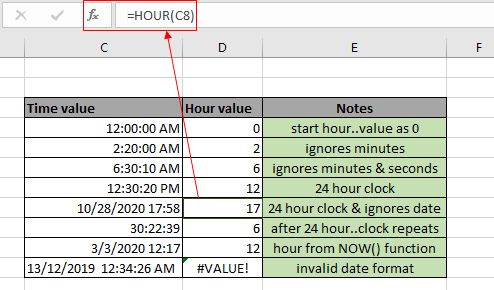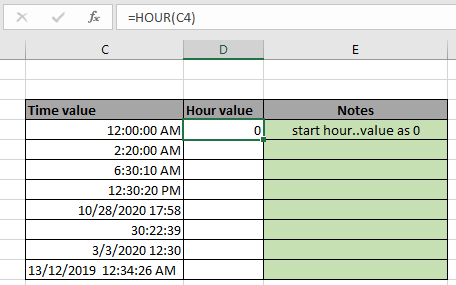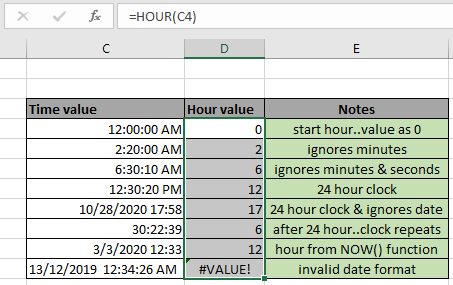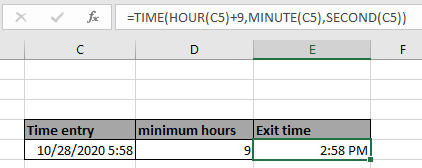# How to use the HOUR function in excelThe Excel HOUR function is a DATE & TIME function. This function takes time value as input and returns the HOUR value from the input time value in numerical format. In this article, we will learn how to use the HOUR function in Excel. Time value in excel is stored as number.

Syntax of HOUR Function:

=HOUR(serial_number)

serial_number : time value

## Example of HOUR Function

All of these might be confusing to understand. So, let's test this formula via running it on the example shown below. So, here I have a table of some data. Excel understands time value as numerical value. The first column contains the time value or date and time value combined, second column contains hour value as result of the using the HOUR function and third column contains the important notes using the function

Use the formula:

=HOUR(C4)It will return 0. Here the argument to the HOUR function is given using cell reference. The returned value is zero as excel considers hour value starting from the 0 as 12:00 AM and 12 as 12:00 PM. As shown in the above snapshot, you can change the format of any cell to any format using the format cell option. Now You can copy the formula from one to the remaining cells using the Ctrl + D shortcut key or using the drag down option in excel.As you can see from the above snapshot that HOUR function takes time value as input and returns the HOUR value from the time value. The function returns #VALUE! Error if the time or date & time value is invalid.

Excel HOUR function : HOUR function with other formulas to return result in HOUR format. HOUR function is mostly used with other date and time functions for calculating HOUR values in

Excel. From one time value extract hour, minute and second for more calculation like the one shown below to get the minimum exit time entry.

Use the formula:

=TIME(HOUR(C5)+9,MINUTE(C5),SECOND(C5))Here the HOUR function extract HOUR value, MINUTE function extracts minute value and second function extracts second value from the given time value and perform operation over numerical values and return the arguments to the TIME function to get in return required time value.

Here are some observational notes shown below.

Notes:

1. The formula only works with numbers.
2. The numerical hour value starts from 12:00 AM as 0 and goes upto 23 as 11:00 PM.
3. Arguments to the function must be numeric values or else the function returns #NAME? Error.
4. No argument to the function returns #NUM! Error.
5. The function ignores minute and second value
6. The function returns #VALUE! Error if the date or time value is invalid.

Hope this article about How to use the HOUR function in Excel is explanatory. Find more articles on DATE & TIME formulas here. If you liked our blogs, share it with your fristarts on Facebook. And also you can follow us on Twitter and Facebook. We would love to hear from you, do let us know how we can improve, complement or innovate our work and make it better for you. Write to us at info@exceltip.com.

Related Article

minutes to hours and minutes | returns a numerical value which represents the same value but in decimal format in place of time format in excel.

Calculate hours between time in Excel | Calculate the hours between two time values in excel. find the time elapsed between end_time & start_time

Time difference in hours as decimal value | Time difference in hours using Excel MOD function and then we need to represent it in the decimal format.

SUM time over 9 hours in Excel | get the sum of time values which are above 9 hours

How to add Decimal Hours to Time | add numeric or decimal time to a time formatted value.

Popular Articles:

50 Excel Shortcuts to Increase Your Productivity | Get faster at your task. These 50 shortcuts will make you work even faster on Excel.

The VLOOKUP Function in Excel | This is one of the most used and popular functions of excel that is used to lookup value from different ranges and sheets.

COUNTIF in Excel 2016 | Count values with conditions using this amazing function. You don't need to filter your data to count specific values. Countif function is essential to prepare your dashboard.

How to Use SUMIF Function in Excel | This is another dashboard essential function. This helps you sum up values on specific conditions.

Terms and Conditions of use

The applications/code on this site are distributed as is and without warranties or liability. In no event shall the owner of the copyrights, or the authors of the applications/code be liable for any loss of profit, any problems or any damage resulting from the use or evaluation of the applications/code.